# NCERT Solutions for Class 7 Maths Chapter 13 – Exponents and Powers

Class 7 Maths NCERT Solutions Chapter 13 – Exponents and Powers comprises of the 4 Exercises

### NCERT Solutions for Class 7 Maths Chapter 13 Exercise 13.1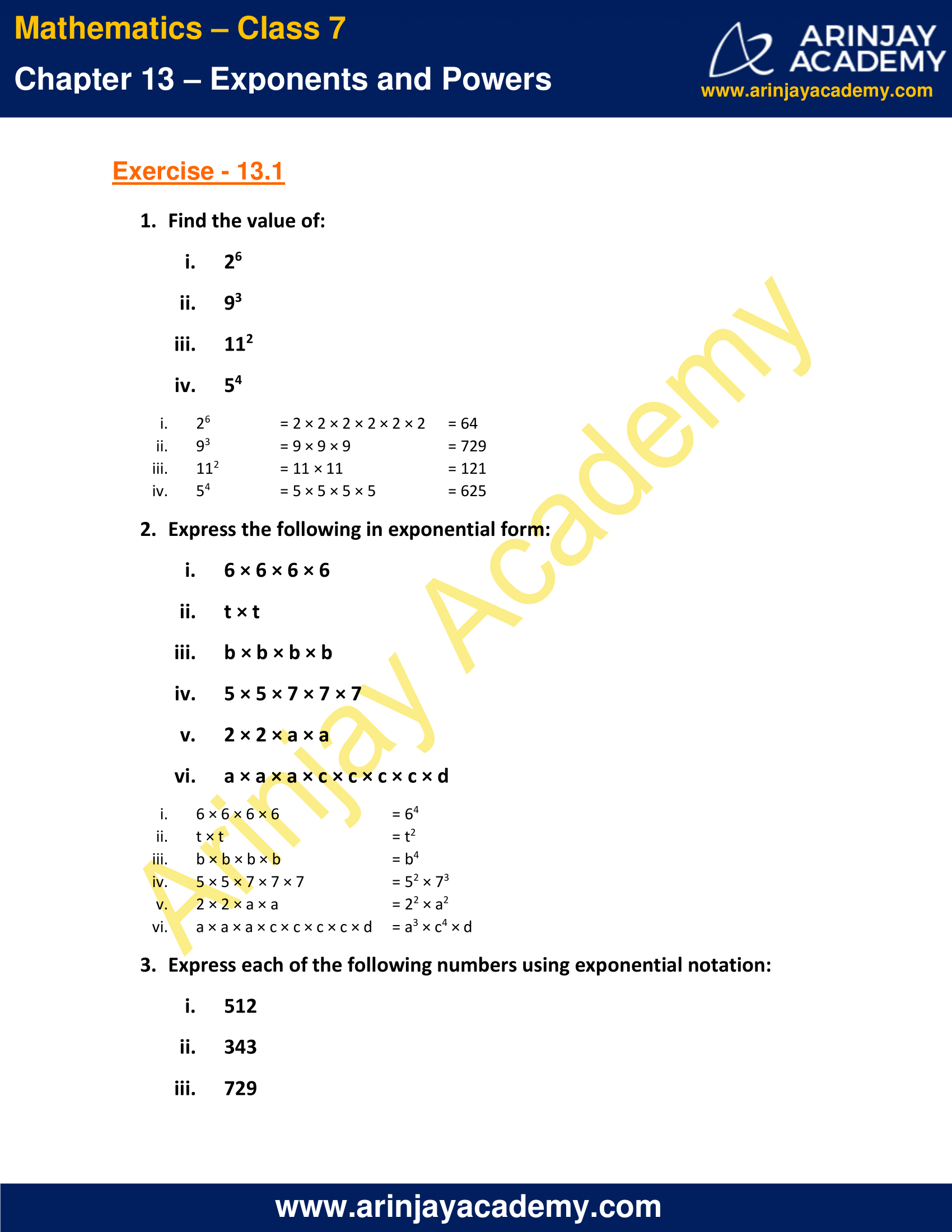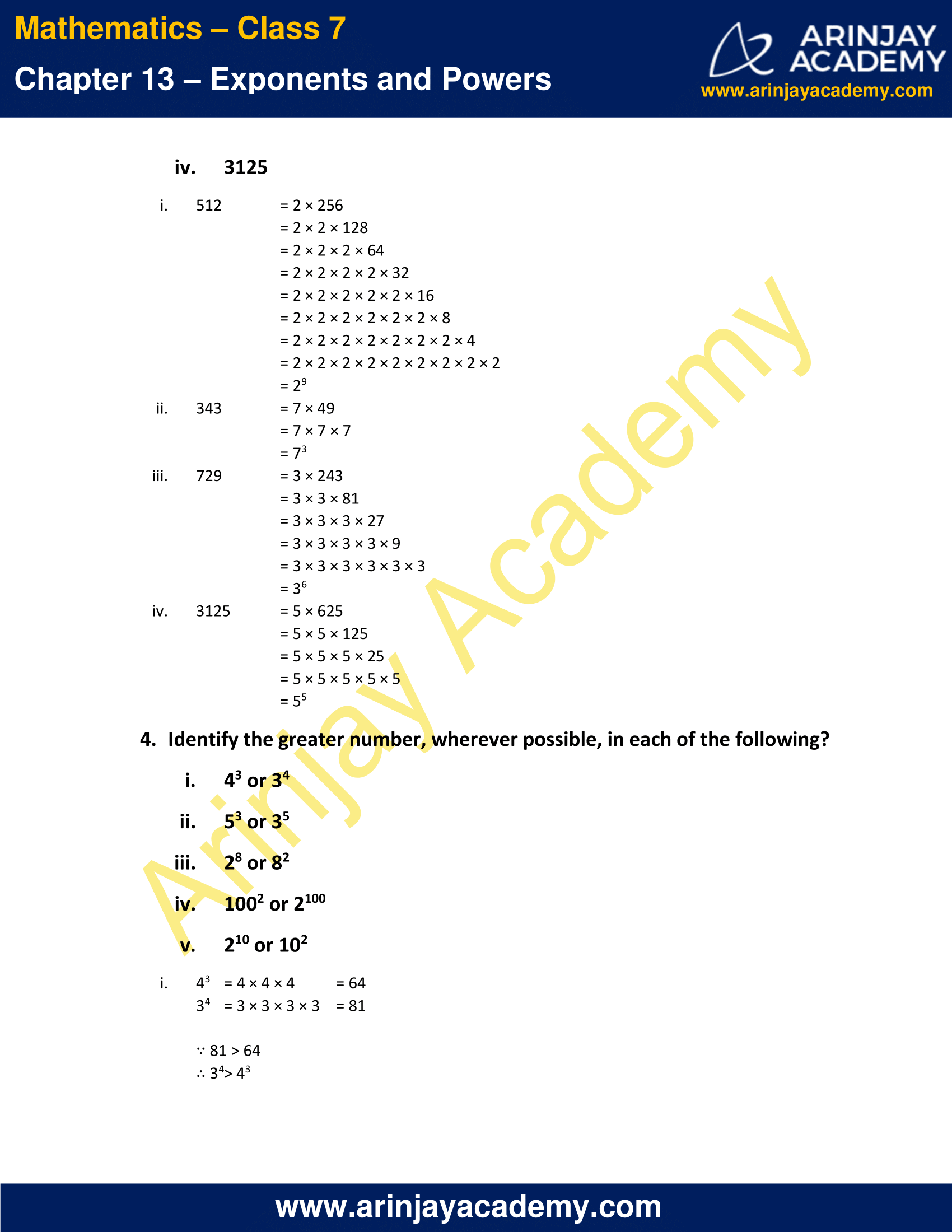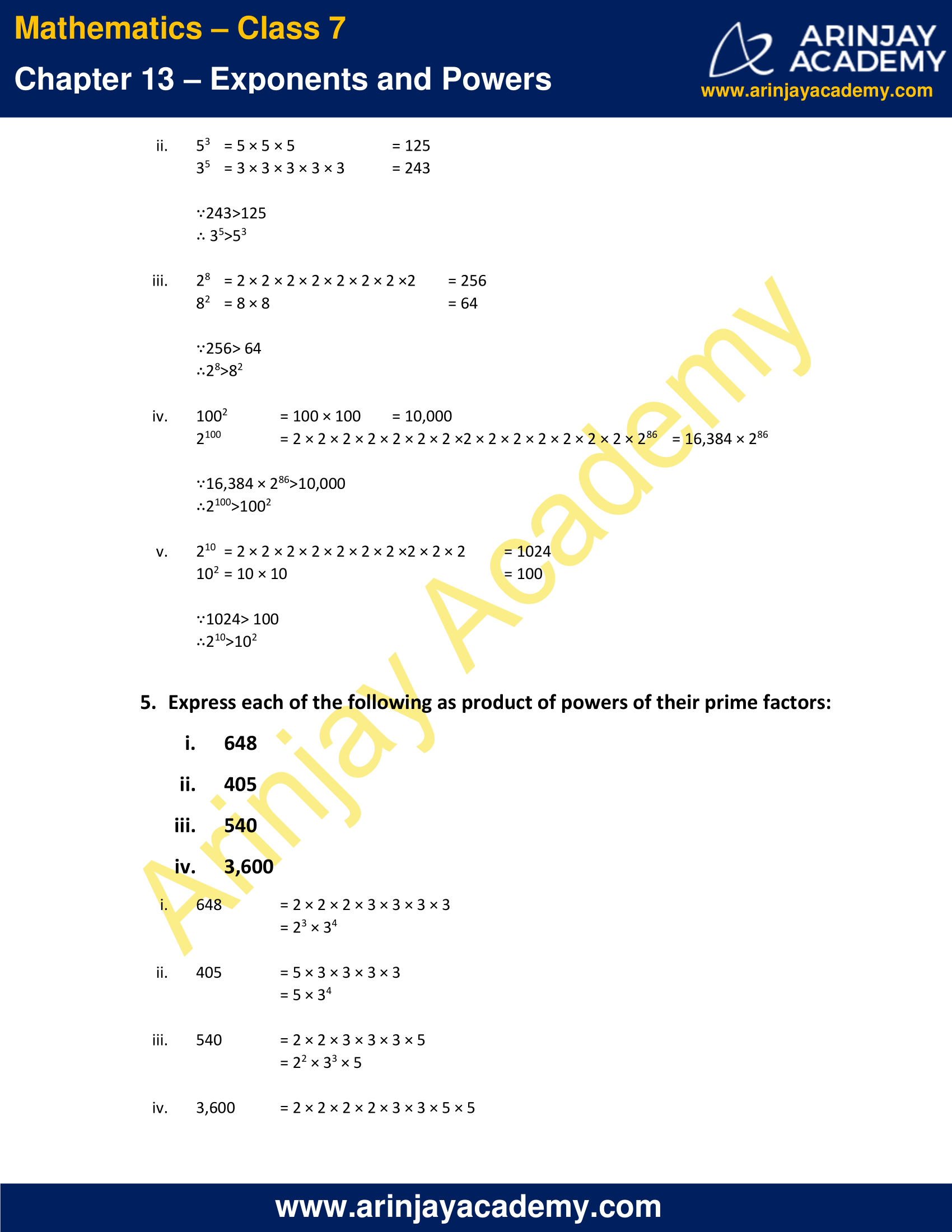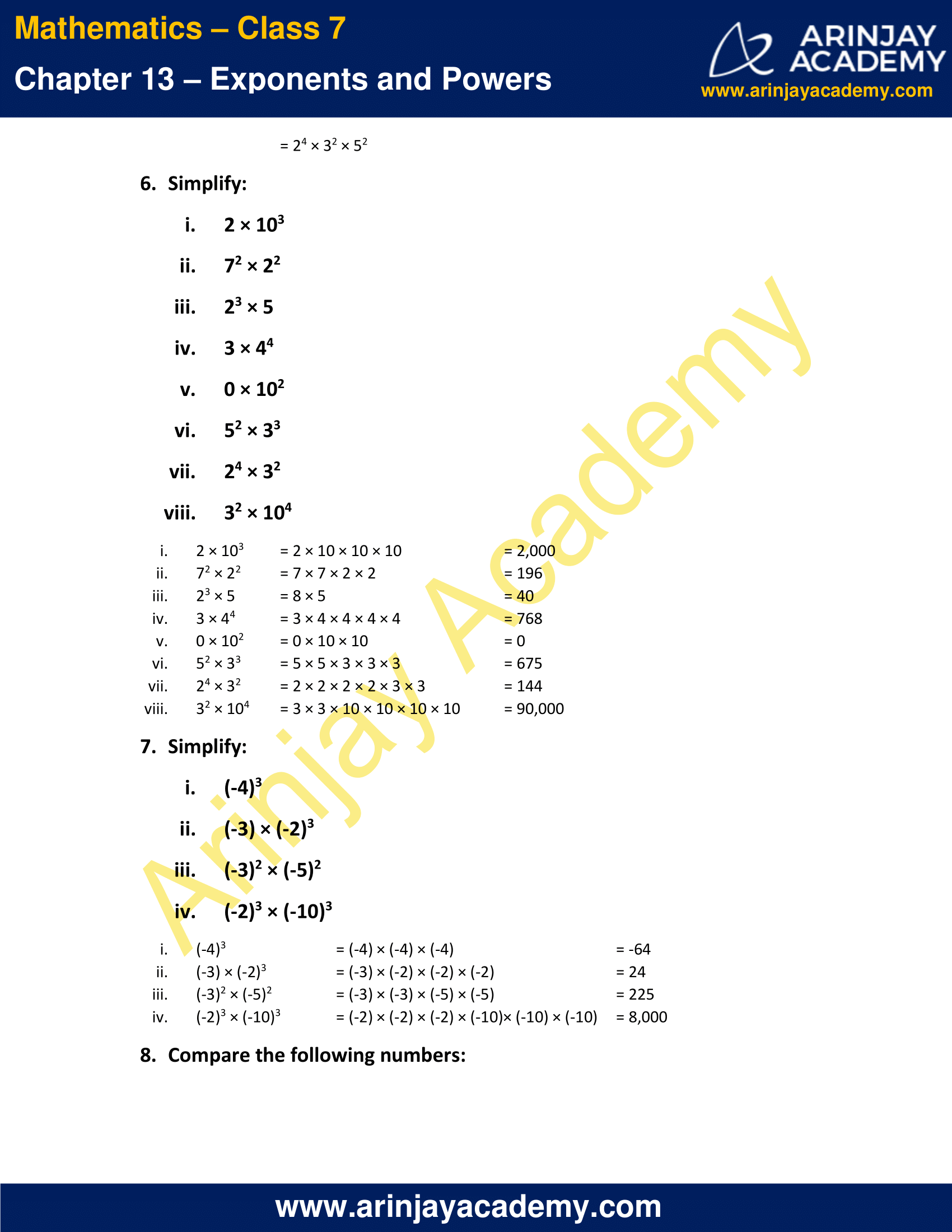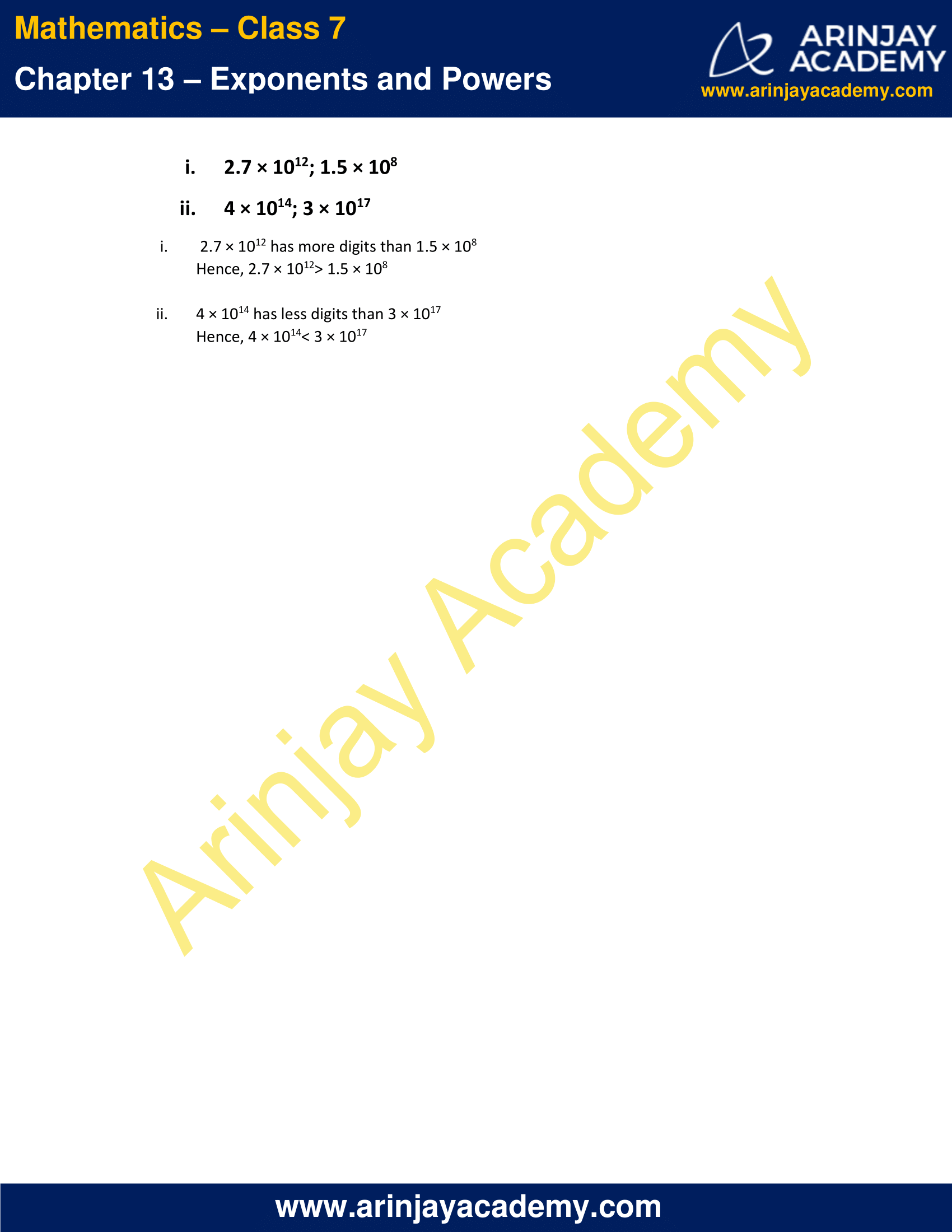### NCERT Solutions for Class 7 Maths Chapter 13 Exercise 13.2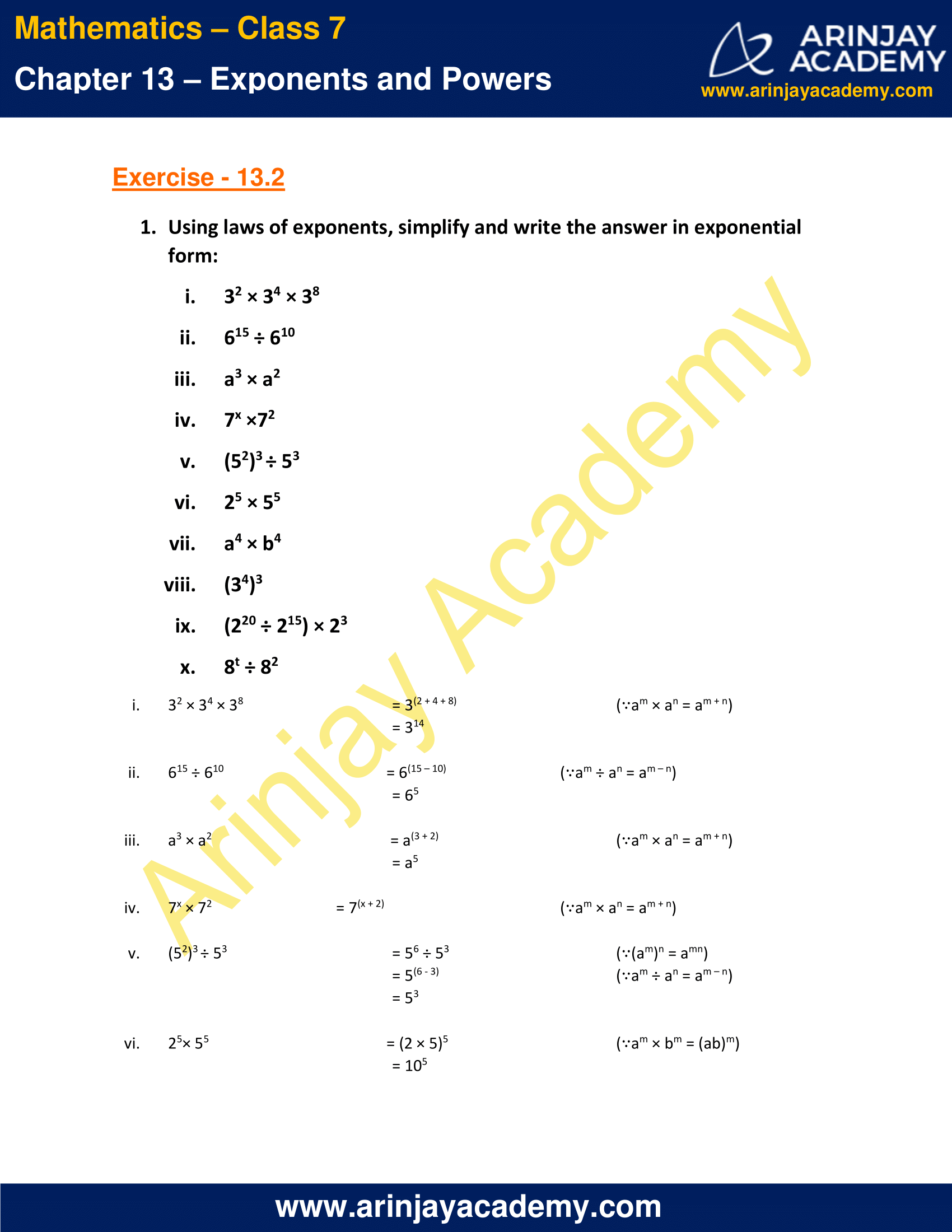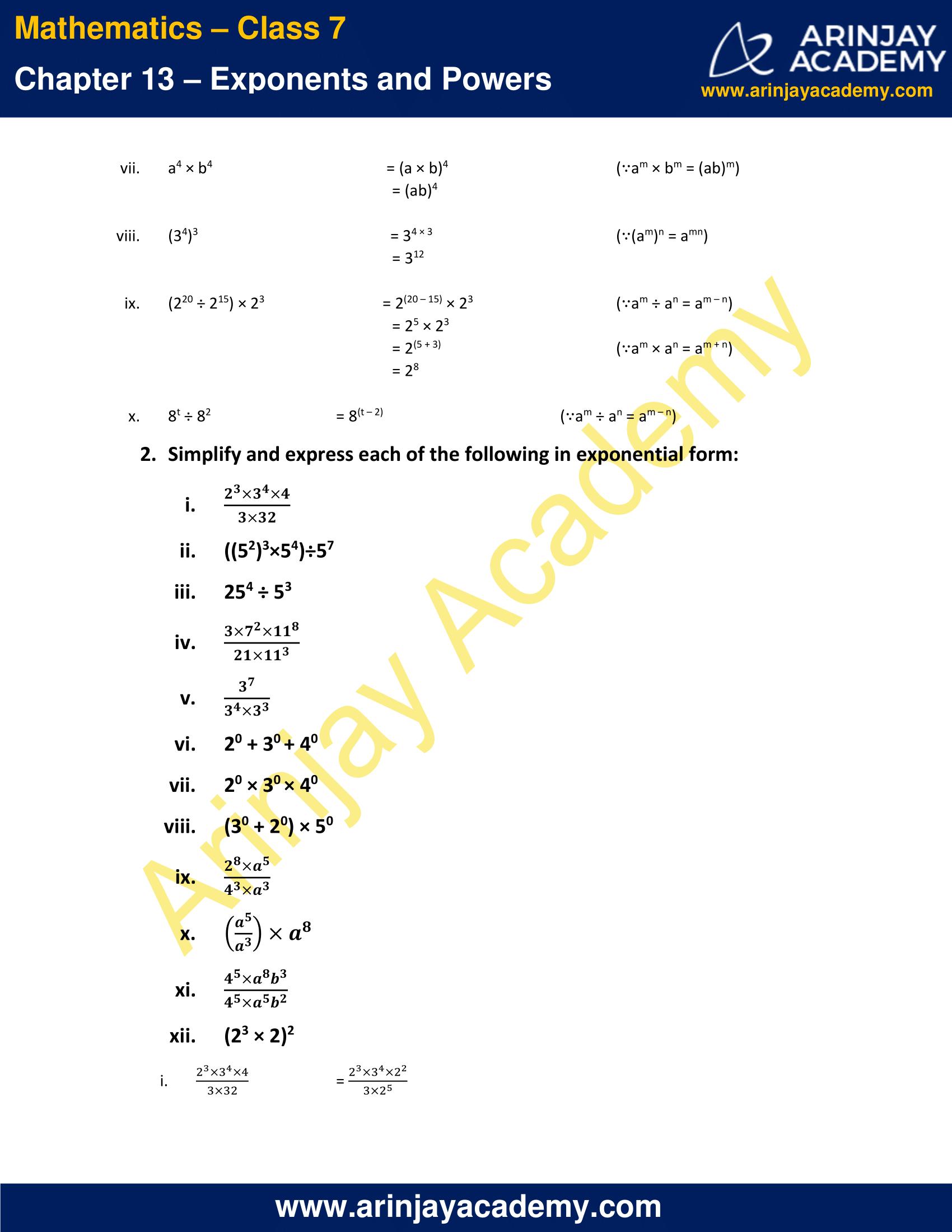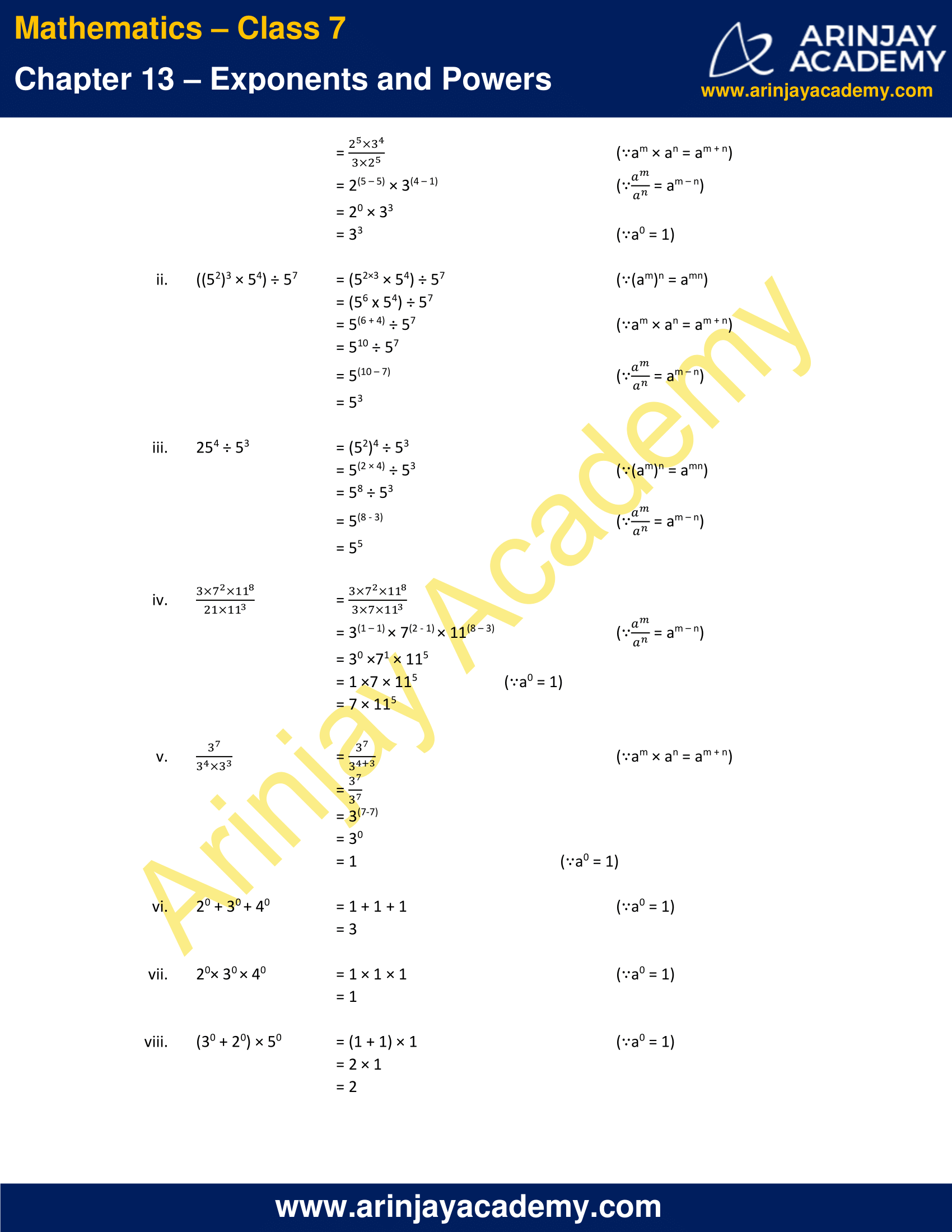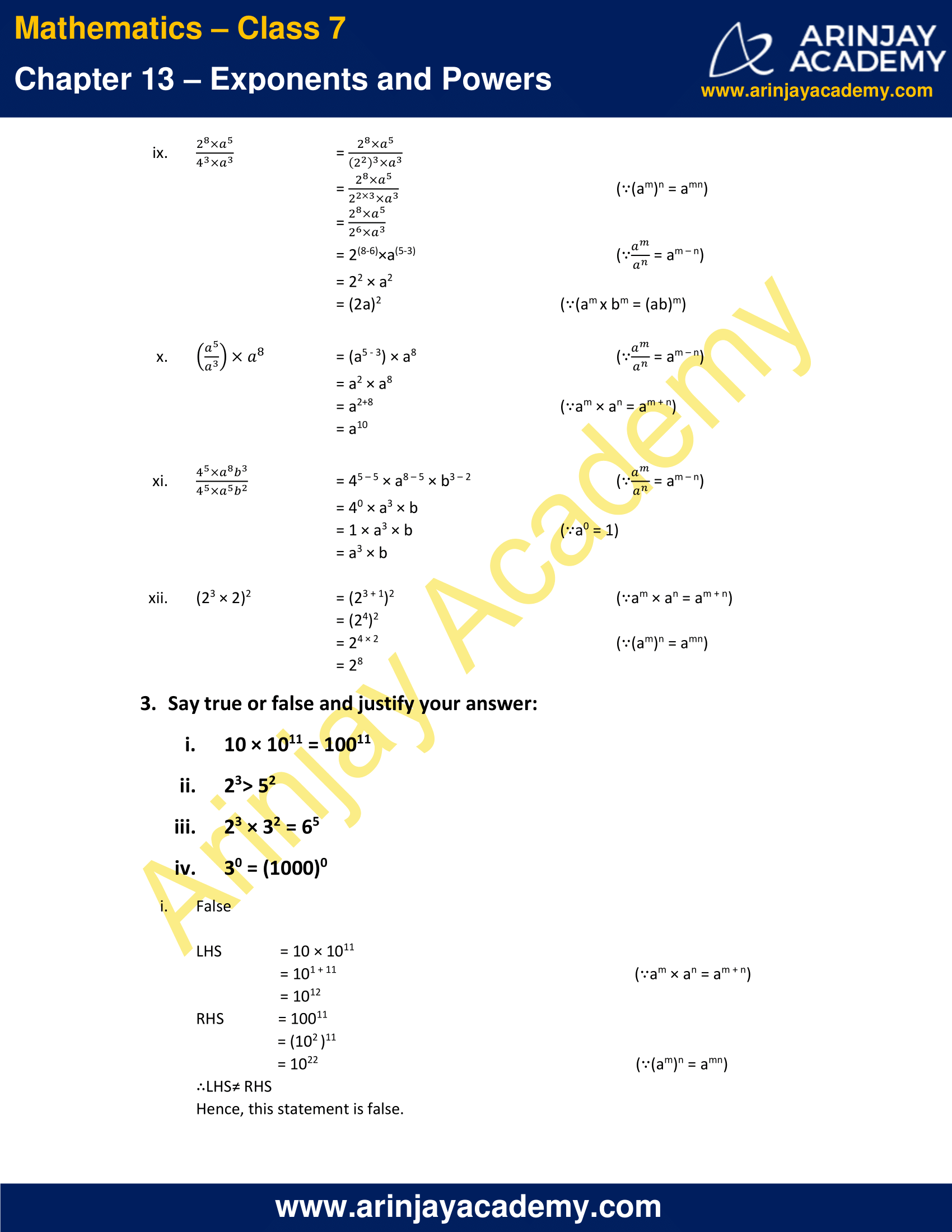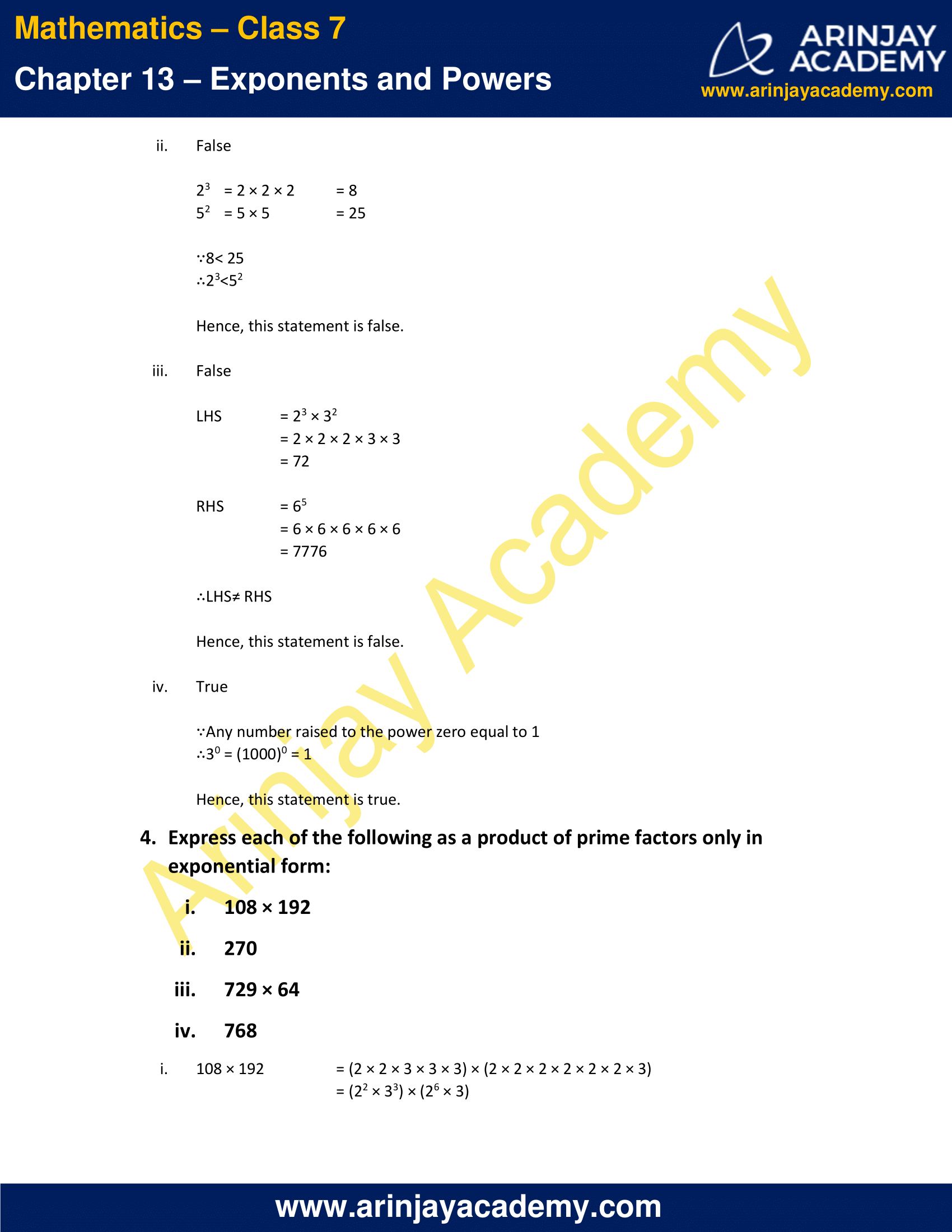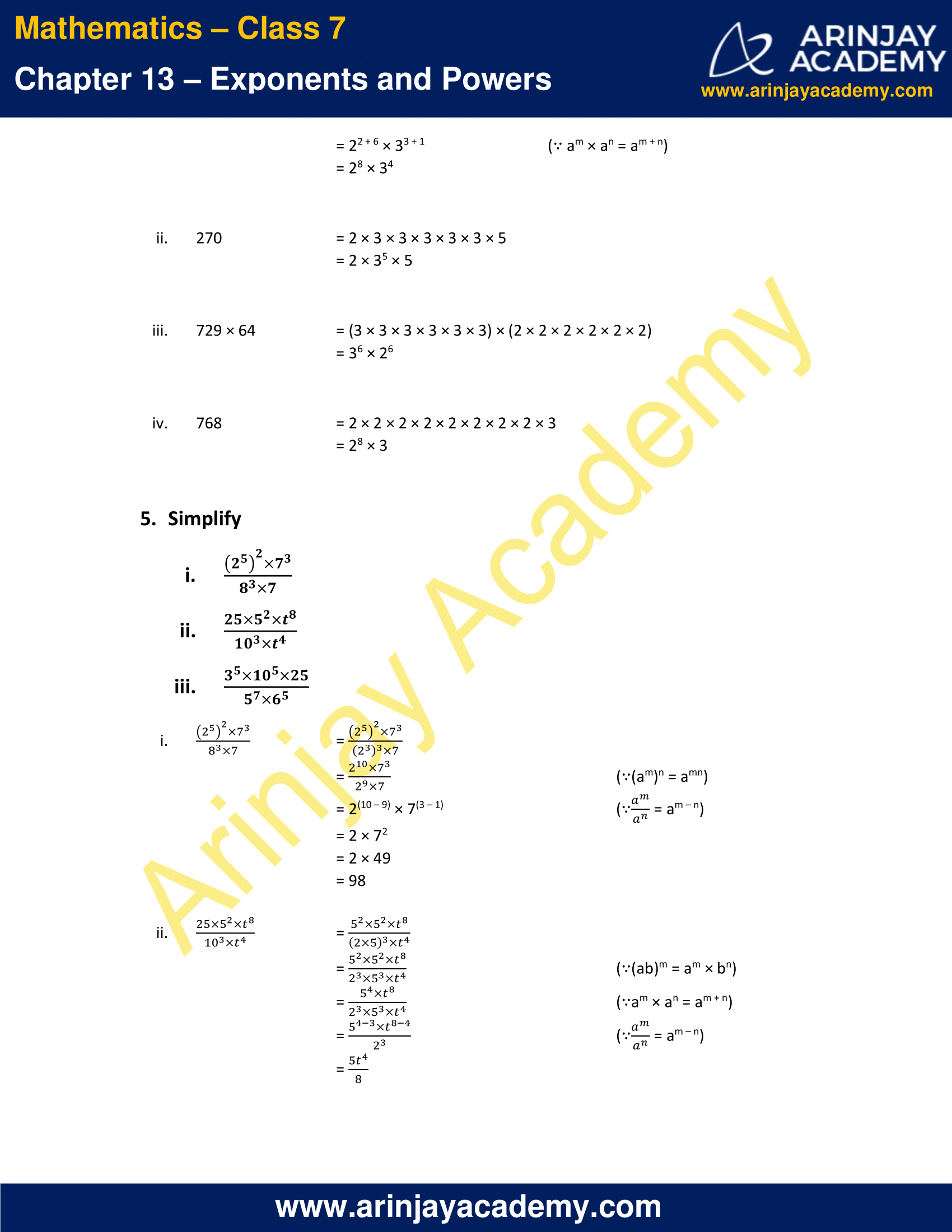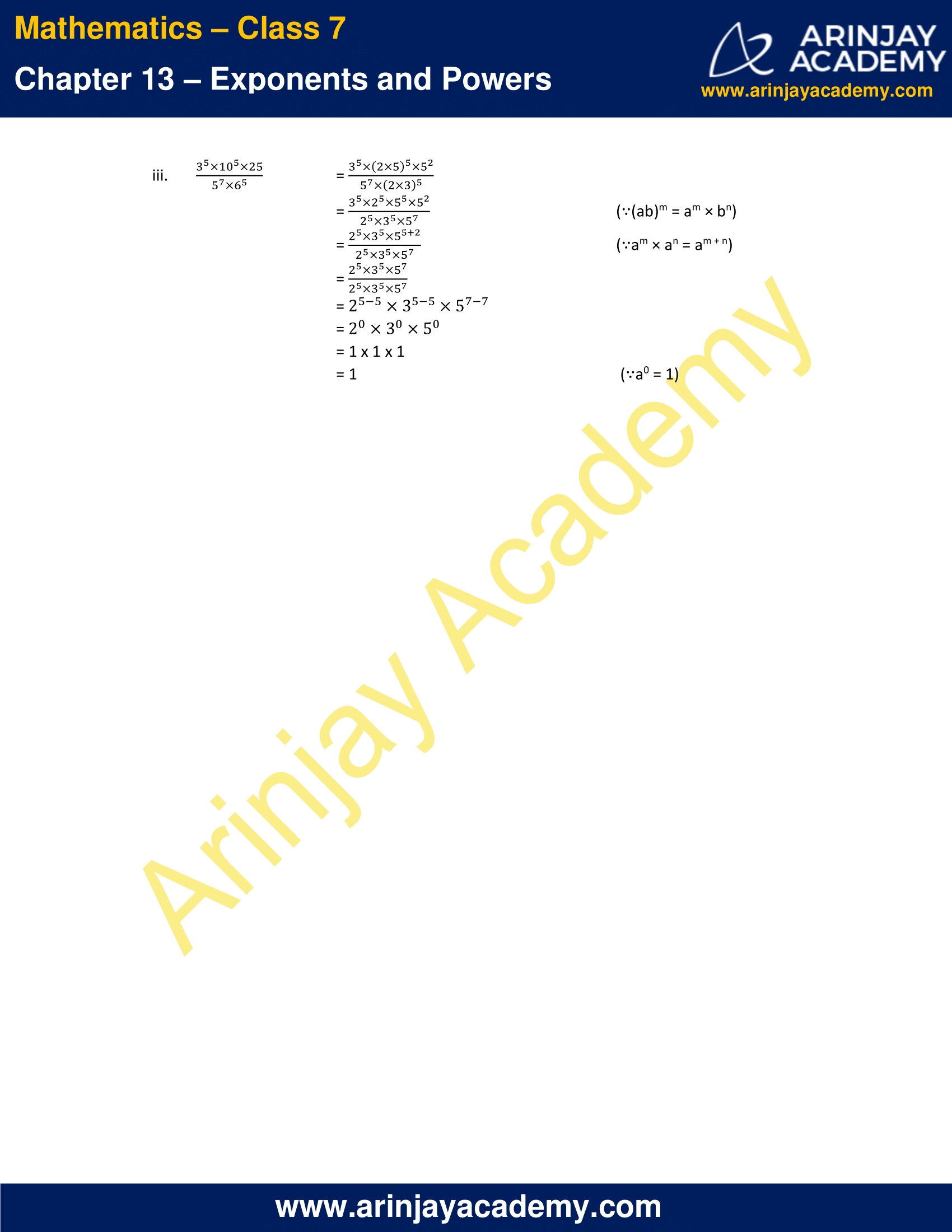### NCERT Solutions for Class 7 Maths Chapter 13 Exercise 13.3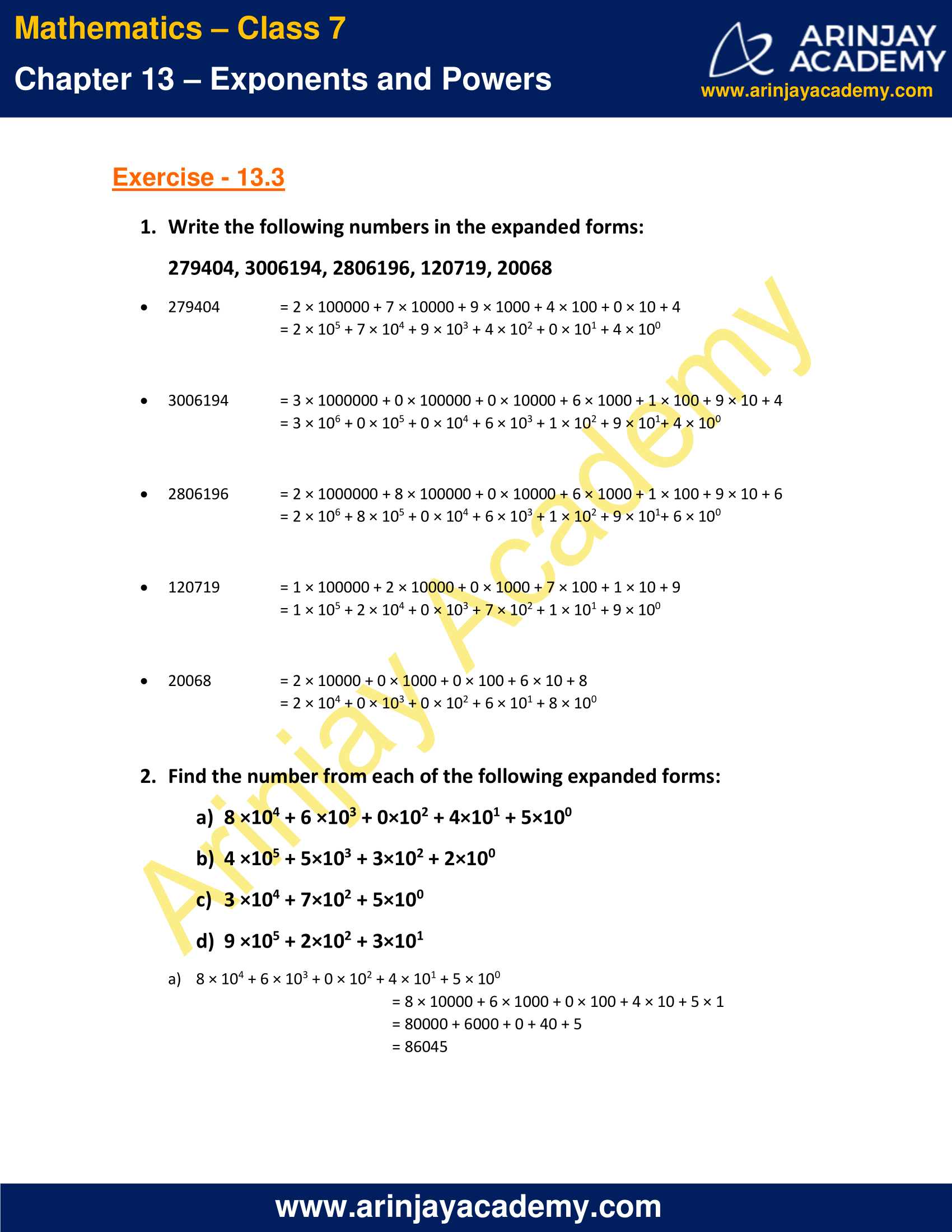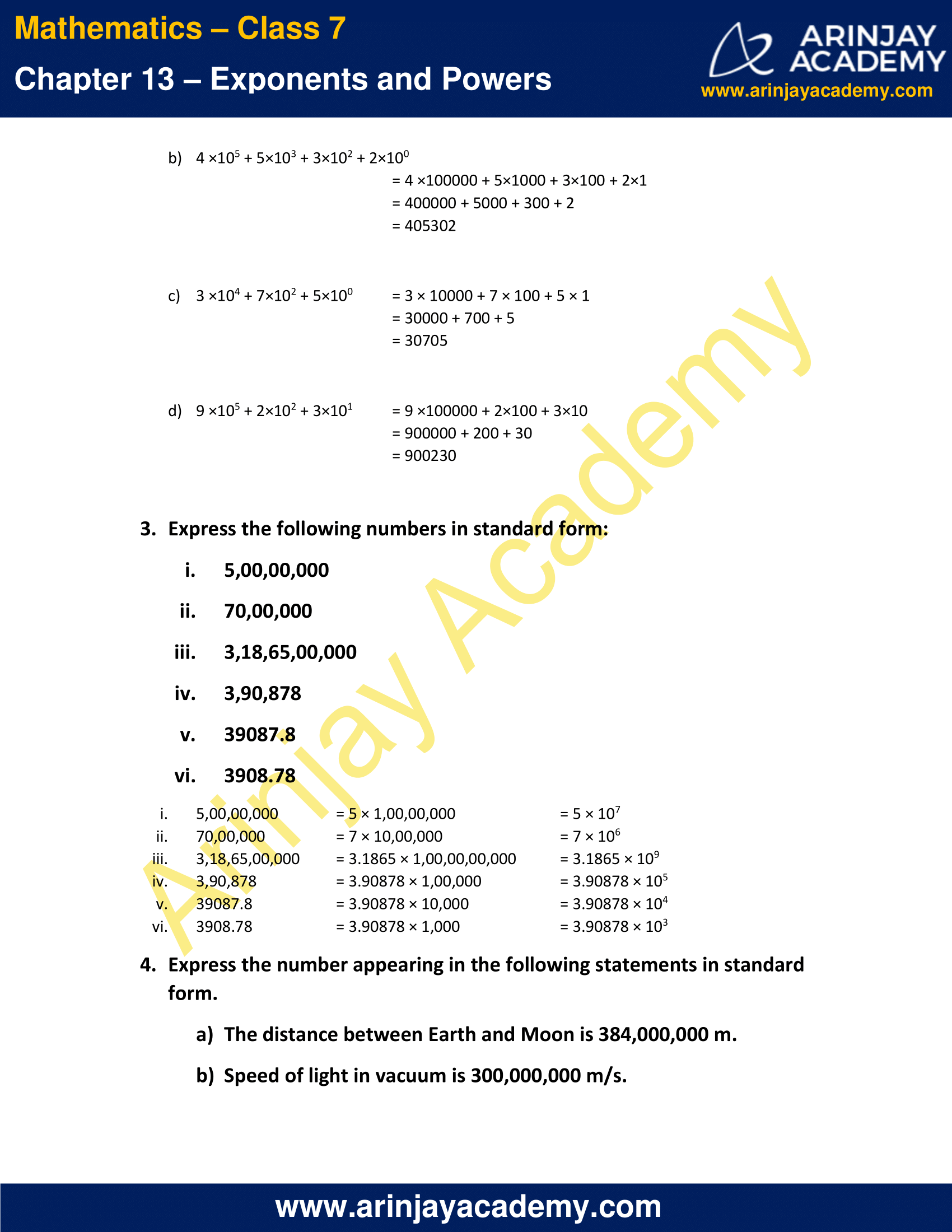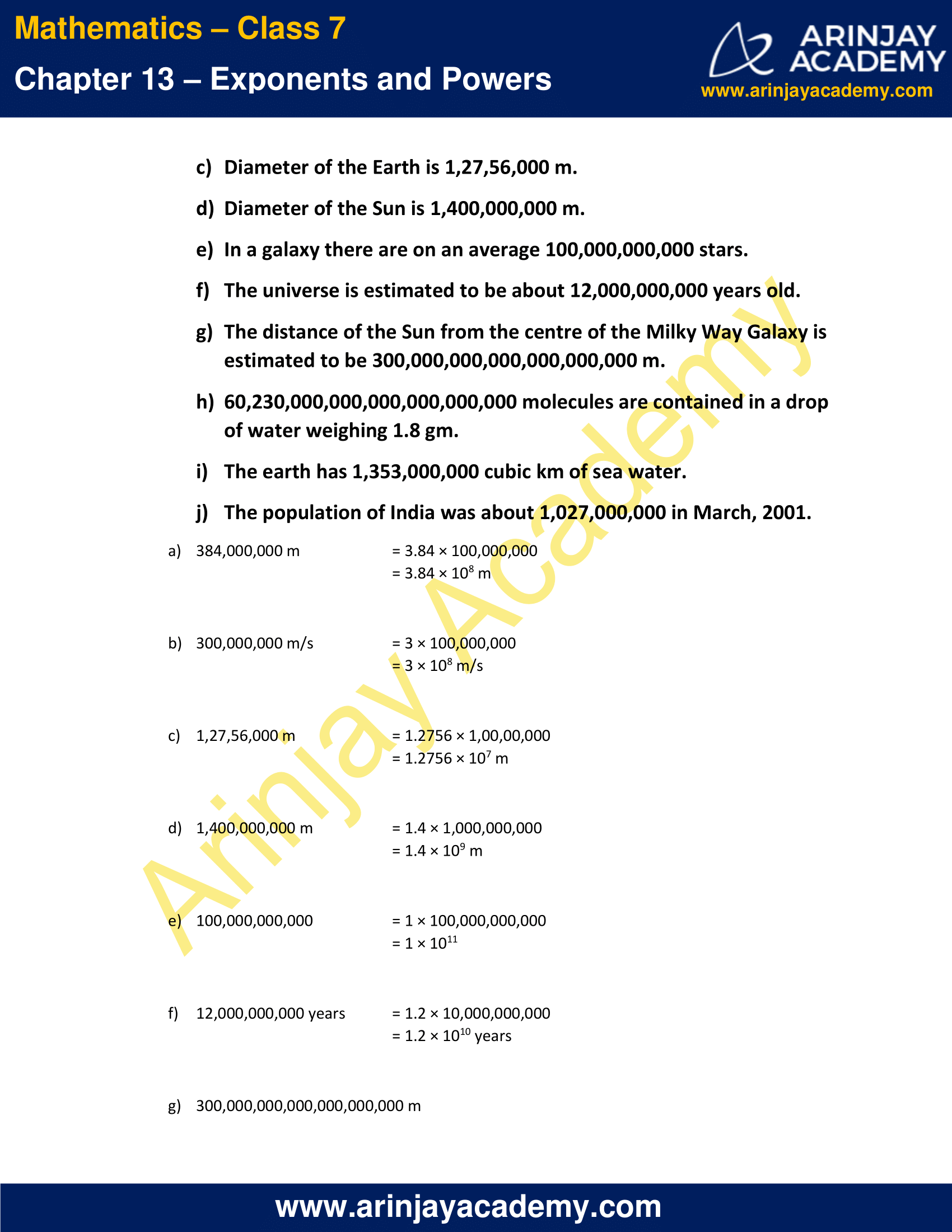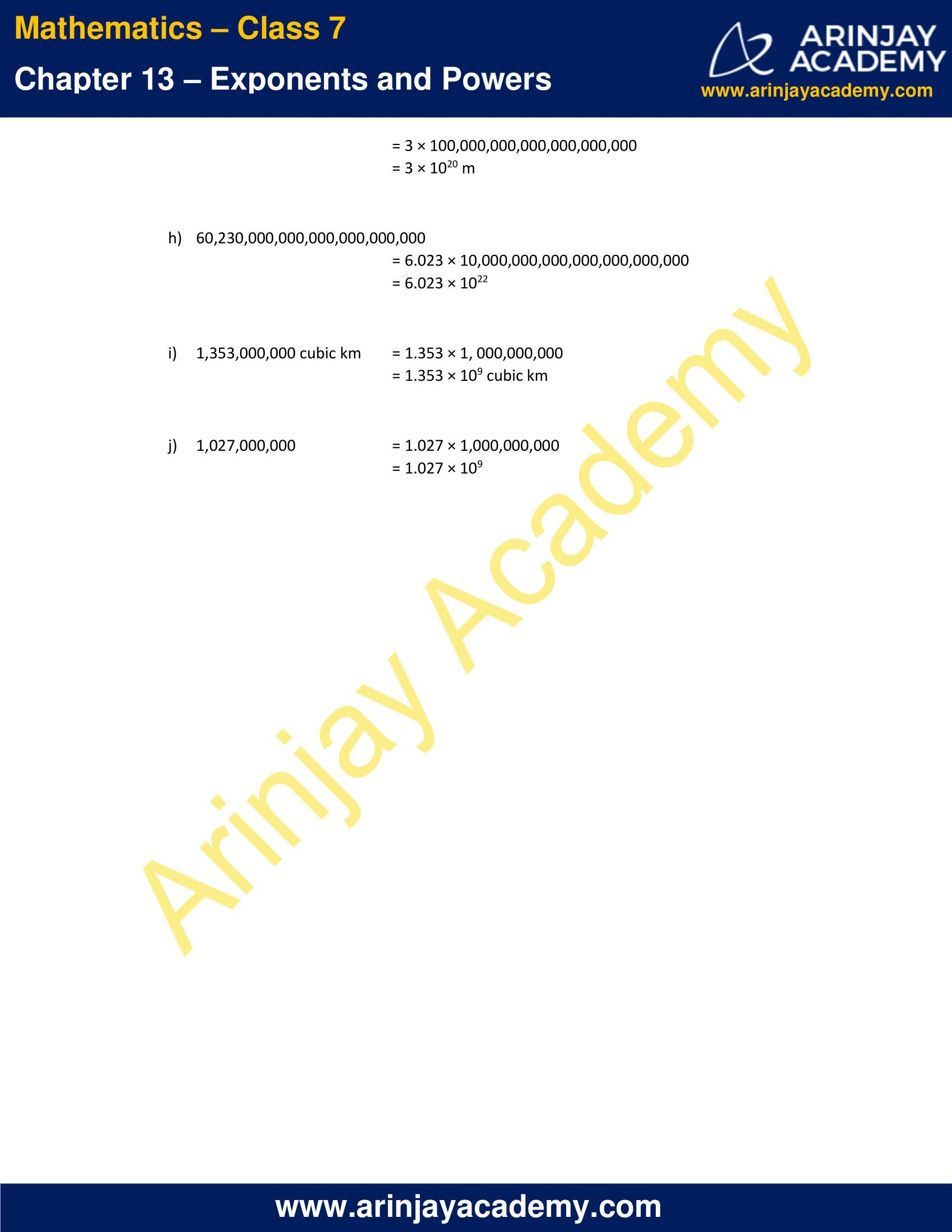### NCERT Solutions for Class 7 Maths Chapter 13 Exercise 13.1

1. Find the value of:

i) 26
ii) 93
iii) 112
iv) 54

i) 26 = 2 × 2 × 2 × 2 × 2 × 2 = 64
ii) 93 = 9 × 9 × 9 = 729
iii) 112 = 11 × 11 = 121
iv) 54 = 5 × 5 × 5 × 5 = 625

2. Express the following in exponential form:

i) 6 × 6 × 6 × 6
ii) t × t
iii) b × b × b × b
iv) 5 × 5 × 7 × 7 × 7

v) 2 × 2 × a × a
vi) a × a × a × c × c × c × c × d

i) 6 × 6 × 6 × 6 = 64
ii) t × t = t2
iii) b × b × b × b = b4
iv) 5 × 5 × 7 × 7 × 7 = 52 × 73
v) 2 × 2 × a × a = 22 × a2
vi) a × a × a × c × c × c × c × d = a3 × c4 × d

3. Express each of the following numbers using exponential notation:

i) 512
ii) 343
iii) 729
iv) 3125

i) 512 = 2 × 256
= 2 × 2 × 128
= 2 × 2 × 2 × 64
= 2 × 2 × 2 × 2 × 32
= 2 × 2 × 2 × 2 × 2 × 16
= 2 × 2 × 2 × 2 × 2 × 2 × 8
= 2 × 2 × 2 × 2 × 2 × 2 × 2 × 4
= 2 × 2 × 2 × 2 × 2 × 2 × 2 × 2 × 2
= 29

ii) 343 = 7 × 49
= 7 × 7 × 7
= 73

iii) 729 = 3 × 243
= 3 × 3 × 81
= 3 × 3 × 3 × 27
= 3 × 3 × 3 × 3 × 9
= 3 × 3 × 3 × 3 × 3 × 3
= 36

iv) 3125 = 5 × 625
= 5 × 5 × 125
= 5 × 5 × 5 × 25
= 5 × 5 × 5 × 5 × 5
= 55

4. Identify the greater number, wherever possible, in each of the following?

i) 43 or 34
ii) 53 or 35
iii) 28 or 82
iv) 1002 or 2100
v) 210 or 102

i) 43 = 4 × 4 × 4 = 64
34    = 3 × 3 × 3 × 3 = 81
∵ 81 > 64
∴ 34 > 43

ii) 53 = 5 × 5 × 5 = 125
35    = 3 × 3 × 3 × 3 × 3 = 243
∵ 243 > 125
∴ 35 > 53

iii) 28 = 2 × 2 × 2 × 2 × 2 × 2 × 2 ×2 = 256
82 = 8 × 8 = 64
∵ 256 > 64
∴ 28 > 82

iv) 1002 = 100 × 100 = 10,000
2100 = 2 × 2 × 2 × 2 × 2 × 2 × 2 ×2 × 2 × 2 × 2 × 2 × 2 × 2 × 286 = 16,384 × 286
∵ 16,384 × 286 > 10,000
∴ 2100 > 1002

v) 210 = 2 × 2 × 2 × 2 × 2 × 2 × 2 ×2 × 2 × 2 = 1024
102 = 10 × 10 = 100
∵ 1024 > 100
∴ 210 > 102

5. Express each of the following as product of powers of their prime factors:

i) 648
ii) 405
iii) 540
iv) 3,600

i) 648 = 2 × 2 × 2 × 3 × 3 × 3 × 3
= 23 × 34

ii) 405 = 5 × 3 × 3 × 3 × 3
= 5 × 34

iii) 540 = 2 × 2 × 3 × 3 × 3 × 5
= 22 × 33 × 5

iv) 3,600 = 2 × 2 × 2 × 2 × 3 × 3 × 5 × 5
= 24 × 32 × 52

6. Simplify:

i) 2 × 103
ii) 72 × 22
iii) 23 × 5

iv) 3 × 44
v) 0 × 102
vi) 52 × 33
vii) 24 × 32
viii) 32 × 104

i) 2 × 103 = 2 × 10 × 10 × 10 = 2,000
ii) 72 × 22 = 7 × 7 × 2 × 2 = 196
iii) 23 × 5 = 8 × 5 = 40
iv) 3 × 44 = 3 × 4 × 4 × 4 × 4 = 768
v) 0 × 102 = 0 × 10 × 10 = 0
vi) 52 × 33 = 5 × 5 × 3 × 3 × 3 = 675
vii) 24 × 32 = 2 × 2 × 2 × 2 × 3 × 3 = 144
viii) 32 × 104 = 3 × 3 × 10 × 10 × 10 × 10 = 90,000

7. Simplify:

i) (-4)3
ii) (-3) × (-2)3
iii) (-3)2 × (-5)2
iv) (-2)3 × (-10)3

i) (-4)3 = (-4) × (-4) × (-4) = -64
ii) (-3) × (-2)3 = (-3) × (-2) × (-2) × (-2) = 24
iii) (-3)2 × (-5)2 = (-3) × (-3) × (-5) × (-5) = 225
iv) (-2)3 × (-10)3 = (-2) × (-2) × (-2) × (-10) × (-10) × (-10) = 8,000

8. Compare the following numbers:

i) 2.7 × 1012; 1.5 × 108
ii) 4 × 1014; 3 × 1017

i) 2.7 × 1012 has more digits than 1.5 × 108
Hence, 2.7 × 1012 > 1.5 × 108

ii) 4 × 1014 has less digits than 3 × 1017
Hence, 4 × 1014 < 3 × 1017

### NCERT Solutions for Class 7 Maths Chapter 13 Exercise 13.2

1. Using laws of exponents, simplify and write the answer in exponential form:

i) 32 × 34 × 38
ii) 615 ÷ 610
iii) a3 × a2
iv) 7x × 72
v) (52)3 ÷ 53
vi) 25 × 55
vii) a4 × b4
viii) (34)3
ix) (220 ÷ 215) × 23
x) 8t ÷ 82

i) 32 × 34 × 38
= 3(2 + 4 + 8)    (∵ am × an = am + n)
= 314

ii) 615 ÷ 610
= 6(15 – 10)    (∵ am ÷ an = am – n)
= 65

iii) a3 × a2
= a(3 + 2)    (∵ am × an = am + n)
= a5

iv) 7x × 72
= 7(x + 2)    (∵ am × an = am + n)

v) (52)3 ÷ 53
= 56 ÷ 53    (∵ (am)n = amn)
= 5(6 – 3)     (∵ am ÷ an = am – n)
= 53

vi) 25 × 55
= (2 × 5)5   (∵ am × bm = (ab)m)
= 105

vii) a4 × b4
= (a × b)4   (∵ am × bm = (ab)m)
= (ab)4

viii) (34)3
= 34 × 3   (∵ (am)n = amn)
= 312

ix) (220 ÷ 215) × 23
= 2(20 – 15) × 23    (∵ am ÷ an = am – n)
= 25 × 23
= 2(5 + 3)   (∵ am × an = am + n)
= 28

x) 8t ÷ 82
= 8(t – 2)   (∵ am ÷ an = am – n)

2. Simplify and express each of the following in exponential form:

i)$\cfrac { 2^{ 3 }\times 3^{ 4 }\times 4 }{ 3\times 32 }$
ii) ((52)3 × 54) ÷ 57
iii) 254 ÷ 53
iv)$\cfrac { 3\times 7^{ 2 }\times 11^{ 8 } }{ 21\times 11^{ 3 } }$
v)$\cfrac { 3^{ 7 } }{ 3^{ 4 }\times 3^{ 3 } }$
vi) 20 + 30 + 40
vii) 20 × 30 × 40
viii) (30 + 20) × 50
ix)$\cfrac { 2^{ 8 }\times a^{ 5 } }{ 4^{ 3 }\times a^{ 3 } }$

x)$\cfrac { a^{ 5 } }{ a^{ 3 } } \times a^{ 8 }$
xi)$\cfrac { 4^{ 5 }\times a^{ 8 }b^{ 3 } }{ 4^{ 5 }\times a^{ 5 }b^{ 2 } }$
xii) (23 × 2)2

i)$\cfrac { 2^{ 3 }\times 3^{ 4 }\times 4 }{ 3\times 32 }$
=$\cfrac { 2^{ 3 }\times 3^{ 4 }\times 2^{ 2 } }{ 3\times 2^{ 5 } }$
=$\cfrac { 2^{ 5 }\times 3^{ 4 } }{ 3\times 2^{ 5 } }$    (∵ am × an = am + n)
= 2(5 – 5) × 3(4 – 1)     (∵$\cfrac { a^{ m } }{ a^{ n } }$ = am – n)
= 20 × 33
= 33    (∵ a0 = 1)

ii) ((52)3 × 54) ÷ 57
= (52×3 × 54) ÷ 57    (∵ (am)n = amn)
= (56 x 54) ÷ 57
= 5(6 + 4) ÷ 57   (∵ am × an = am + n)
= 510 ÷ 57
= 5(10 – 7)  (∵$\cfrac { a^{ m } }{ a^{ n } }$ = am – n)
= 53

iii) 254 ÷ 53 = (52)4 ÷ 53
= 5(2 × 4) ÷ 53   (∵ (am)n = amn)
= 58 ÷ 53
= 5(8 – 3)  (∵$\cfrac { a^{ m } }{ a^{ n } }$ = am – n)
= 55

iv)$\cfrac { 3\times 7^{ 2 }\times 11^{ 8 } }{ 21\times 11^{ 3 } }$
=$\cfrac { 3\times 7^{ 2 }\times 11^{ 8 } }{ 3\times 7\times 11^{ 3 } }$
= 3(1 – 1) × 7(2 – 1) × 11(8 – 3)  (∵$\cfrac { a^{ m } }{ a^{ n } }$ = am – n)
= 30 ×71 × 115
= 1 ×7 × 115      (∵ a0 = 1)
= 7 × 115

v)$\cfrac { 3^{ 7 } }{ 3^{ 4 }\times 3^{ 3 } }$
=$\cfrac { 3^{ 7 } }{ 3^{ (4+3) } }$     (∵ am × an = am + n)
=$\cfrac { 3^{ 7 } }{ 3^{ 7 } }$
= 3(7-7)
= 30
= 1   (∵ a0 = 1)

vi) 20 + 30 + 40
= 1 + 1 + 1   (∵ a0 = 1)
= 3

vii) 20 × 30 × 40
= 1 × 1 × 1     (∵ a0 = 1)
= 1

viii) (30 + 20) × 50
= (1 + 1) × 1    (∵ a0 = 1)
= 2 × 1
= 2

ix)$\cfrac { 2^{ 8 }\times a^{ 5 } }{ 4^{ 3 }\times a^{ 3 } }$
=$\cfrac { 2^{ 8 }\times a^{ 5 } }{ (2^{ 2 })^{ 3 }\times a^{ 3 } }$
=$\cfrac { 2^{ 8 }\times a^{ 5 } }{ 2^{ (2\times 3) }\times a^{ 3 } }$  (∵ (am)n = amn)
=$\cfrac { 2^{ 8 }\times a^{ 5 } }{ 2^{ 6 }\times a^{ 3 } }$
= 2(8-6)×a(5-3)  (∵$\cfrac { a^{ m } }{ a^{ n } }$ = am – n)
= 22 × a2
= (2a)2     (∵ (am x bm = (ab)m)

x)$\cfrac { a^{ 5 } }{ a^{ 3 } } \times a^{ 8 }$
= (a5 – 3) × a8 (∵$\cfrac { a^{ m } }{ a^{ n } }$ = am – n)
= a2 × a8
= a2+8   (∵ am × an = am + n)
= a10

xi)$\cfrac { 4^{ 5 }\times a^{ 8 }b^{ 3 } }{ 4^{ 5 }\times a^{ 5 }b^{ 2 } }$
= 45 – 5 × a8 – 5 × b3 – 2  (∵$\cfrac { a^{ m } }{ a^{ n } }$ = am – n)
= 40 × a3 × b
= 1 × a3 × b    (∵ a0 = 1)
= a3 × b

xii) (23 × 2)2
= (23 + 1)2   (∵ am × an = am + n)
= (24)2
= 24 × 2  (∵ (am)n = amn)
= 28

i) 10 × 1011 = 10011
ii) 23 > 52
iii) 23 × 32 = 65
iv) 30 = (1000)0

i) False
LHS = 10 × 1011
= 101 + 11   (∵ am × an = am + n)
= 1012
RHS = 10011
= (102 )11
= 1022     (∵ (am)n = amn)
∴ LHS ≠ RHS
Hence, this statement is false.

ii) False
23 = 2 × 2 × 2 = 8
52 = 5 × 5 = 25
∵ 8 < 25
∴ 23 < 52
Hence, this statement is false.

iii) False
LHS = 23 × 32
= 2 × 2 × 2 × 3 × 3
= 72
RHS = 65
= 6 × 6 × 6 × 6 × 6
= 7776
∴ LHS ≠ RHS
Hence, this statement is false.

iv) True
∵ Any number raised to the power zero equal to 1
∴ 30 = (1000)0 = 1
Hence, this statement is true.

4. Express each of the following as a product of prime factors only in exponential form:

i) 108 × 192
ii) 270
iii) 729 × 64
iv) 768

i) 108 × 192 = (2 × 2 × 3 × 3 × 3) × (2 × 2 × 2 × 2 × 2 × 2 × 3)
= (22 × 33) × (26 × 3)
= 22 + 6 × 33 + 1    (∵ am × an = am + n)
= 28 × 34

ii) 270 = 2 × 3 × 3 × 3 × 3 × 3 × 5
= 2 × 35 × 5

iii) 729 × 64 = (3 × 3 × 3 × 3 × 3 × 3) × (2 × 2 × 2 × 2 × 2 × 2)
= 36 × 26

iv) 768 = 2 × 2 × 2 × 2 × 2 × 2 × 2 × 2 × 3
= 28 × 3

5. Simplify

i)$\cfrac { (2^{ 5 })^{ 2 }\times 7^{ 3 } }{ 8^{ 3 }\times 7 }$
ii)$\cfrac { 25\times 5^{ 2 }\times t^{ 8 } }{ 10^{ 3 }\times t^{ 4 } }$
iii)$\cfrac { 3^{ 5 }\times 10^{ 5 }\times 25 }{ 5^{ 7 }\times 6^{ 5 } }$

i)$\cfrac { (2^{ 5 })^{ 2 }\times 7^{ 3 } }{ 8^{ 3 }\times 7 }$
=$\cfrac { (2^{ 5 })^{ 2 }\times 7^{ 3 } }{ (2^{ 3 })^{ 3 }\times 7 }$
=$\cfrac { 2^{ 10 }\times 7^{ 3 } }{ 2^{ 9 }\times 7 }$     (∵ (am)n = amn)
= 2(10 – 9) × 7(3 – 1)    (∵$\cfrac { a^{ m } }{ a^{ n } }$ = am – n)
= 2 × 72
= 2 × 49
= 98

ii)$\cfrac { 25\times 5^{ 2 }\times t^{ 8 } }{ 10^{ 3 }\times t^{ 4 } }$
=$\cfrac { 5^{ 2 }\times 5^{ 2 }\times t^{ 8 } }{ (2\times 5)^{ 3 }\times t^{ 4 } }$
=$\cfrac { 5^{ 2 }\times 5^{ 2 }\times t^{ 8 } }{ 2^{ 3 }\times 5^{ 3 }\times t^{ 4 } }$    (∵ (ab)m = am × bn)
=$\cfrac { 5^{ 4 }\times t^{ 8 } }{ 2^{ 3 }\times 5^{ 3 }\times t^{ 4 } }$   (∵ am × an = am + n)
=$\cfrac { 5^{ (4-3) }\times t^{ (8-4) } }{ 2^{ 3 } }$  (∵$\cfrac { a^{ m } }{ a^{ n } }$ = am – n)
=$\cfrac { 5t^{ 4 } }{ 8 }$

iii)$\cfrac { 3^{ 5 }\times 10^{ 5 }\times 25 }{ 5^{ 7 }\times 6^{ 5 } }$
=$\cfrac { 3^{ 5 }\times (2\times 5)^{ 5 }\times 5^{ 2 } }{ 5^{ 7 }\times (2\times 3)^{ 5 } }$
=$\cfrac { 3^{ 5 }\times 2^{ 5 }\times 5^{ 5 }\times 5^{ 2 } }{ 2^{ 5 }\times 3^{ 5 }\times 5^{ 7 } }$   (∵ (ab)m = am × bn)
=$\cfrac { 2^{ 5 }\times 3^{ 5 }\times 5^{ (5+2) } }{ 2^{ 5 }\times 3^{ 5 }\times 5^{ 7 } }$   (∵ am × an = am + n)
=$\cfrac { 2^{ 5 }\times 3^{ 5 }\times 5^{ 7 } }{ 2^{ 5 }\times 3^{ 5 }\times 5^{ 7 } }$
=$2^{ (5-5) }\times 3^{ (5-5) }\times 5^{ (7-7) }$
=$2^{ 0 }\times 3^{ 0 }\times 5^{ 0 }$
= 1 x 1 x 1
= 1     (∵ a0 = 1)

### NCERT Solutions for Class 7 Maths Chapter 13 Exercise 13.3

1. Write the following numbers in the expanded forms:
279404, 3006194, 2806196, 120719, 20068

• 279404 = 2 × 100000 + 7 × 10000 + 9 × 1000 + 4 × 100 + 0 × 10 + 4
= 2 × 105 + 7 × 104 + 9 × 103 + 4 × 102 + 0 × 101 + 4 × 100
• 3006194 = 3 × 1000000 + 0 × 100000 + 0 × 10000 + 6 × 1000 + 1 × 100 + 9 × 10 + 4
= 3 × 106 + 0 × 105 + 0 × 104 + 6 × 103 + 1 × 102 + 9 × 101 + 4 × 100
• 2806196 = 2 × 1000000 + 8 × 100000 + 0 × 10000 + 6 × 1000 + 1 × 100 + 9 × 10 + 6
= 2 × 106 + 8 × 105 + 0 × 104 + 6 × 103 + 1 × 102 + 9 × 101 + 6 × 100
• 120719 = 1 × 100000 + 2 × 10000 + 0 × 1000 + 7 × 100 + 1 × 10 + 9
= 1 × 105 + 2 × 104 + 0 × 103 + 7 × 102 + 1 × 101 + 9 × 100
• 20068 = 2 × 10000 + 0 × 1000 + 0 × 100 + 6 × 10 + 8
= 2 × 104 + 0 × 103 + 0 × 102 + 6 × 101 + 8 × 100

2. Find the number from each of the following expanded forms:

a) 8 × 104 + 6 × 103 + 0 × 102 + 4 × 101 + 5 × 100
b) 4 × 105 + 5 × 103 + 3 × 102 + 2 × 100
c) 3 × 104 + 7 × 102 + 5 × 100
d) 9 × 105 + 2 × 102 + 3 × 101

a) 8 × 104 + 6 × 103 + 0 × 102 + 4 × 101 + 5 × 100
= 8 × 10000 + 6 × 1000 + 0 × 100 + 4 × 10 + 5 × 1
= 80000 + 6000 + 0 + 40 + 5
= 86045

b) 4 × 105 + 5 × 103 + 3 × 102 + 2 × 100
= 4 × 100000 + 5 × 1000 + 3 × 100 + 2 × 1
= 400000 + 5000 + 300 + 2
= 405302

c) 3 × 104 + 7 × 102 + 5 × 100 = 3 × 10000 + 7 × 100 + 5 × 1
= 30000 + 700 + 5
= 30705

d) 9 × 105 + 2 × 102 + 3 × 101 = 9 × 100000 + 2 × 100 + 3 × 10
= 900000 + 200 + 30
= 900230

3. Express the following numbers in standard form:

i) 5,00,00,000
ii) 70,00,000
iii) 3,18,65,00,000
iv) 3,90,878
v) 39087.8
vi) 3908.78

i) 5,00,00,000 = 5 × 1,00,00,000 = 5 × 107
ii) 70,00,000 = 7 × 10,00,000 = 7 × 106
iii) 3,18,65,00,000 = 3.1865 × 1,00,00,00,000 = 3.1865 × 109
iv) 3,90,878 = 3.90878 × 1,00,000 = 3.90878 × 105
v) 39087.8 = 3.90878 × 10,000 = 3.90878 × 104
vi) 3908.78 = 3.90878 × 1,000 = 3.90878 × 103

4. Express the number appearing in the following statements in standard form.

a) The distance between Earth and Moon is 384,000,000 m.
b) Speed of light in vacuum is 300,000,000 m/s.
c) Diameter of the Earth is 1,27,56,000 m.
d) Diameter of the Sun is 1,400,000,000 m.
e) In a galaxy there are on an average 100,000,000,000 stars.
f) The universe is estimated to be about 12,000,000,000 years old.
g) The distance of the Sun from the centre of the Milky Way Galaxy is estimated to be 300,000,000,000,000,000,000 m.
h) 60,230,000,000,000,000,000,000 molecules are contained in a drop of water weighing 1.8 gm.
i) The earth has 1,353,000,000 cubic km of sea water.
j) The population of India was about 1,027,000,000 in March, 2001.

a) 384,000,000 m
= 3.84 × 100,000,000
= 3.84 × 108 m

b) 300,000,000 m/s
= 3 × 100,000,000
= 3 × 108 m/s

c) 1,27,56,000 m
= 1.2756 × 1,00,00,000
= 1.2756 × 107 m

d) 1,400,000,000 m
= 1.4 × 1,000,000,000
= 1.4 × 109 m

e) 100,000,000,000
= 1 × 100,000,000,000
= 1 × 1011

f) 12,000,000,000 years
= 1.2 × 10,000,000,000
= 1.2 × 1010 years

g) 300,000,000,000,000,000,000 m
= 3 × 100,000,000,000,000,000,000
= 3 × 1020 m

h) 60,230,000,000,000,000,000,000
= 6.023 × 10,000,000,000,000,000,000,000
= 6.023 × 1022

i) 1,353,000,000 cubic km
= 1.353 × 1, 000,000,000
= 1.353 × 109 cubic km

j) 1,027,000,000
= 1.027 × 1,000,000,000
= 1.027 × 109

This Chapter contains the Exercises relating to the following topics , which are discussed in Chapter 13 – Exponents and Powers Class 7 NCERT book  : –

• 13.1 INTRODUCTION
• 13.2 EXPONENTS
• 13.3 LAWS OF EXPONENTS
• 13.3.1 Multiplying Powers with the Same Base
• 13.3.2 Dividing Powers with the Same Base
• 13.3.3 Taking Power of a Power
• 13.3.4 Multiplying Powers with the Same Exponents
• 13.3.5 Dividing Powers with the Same Exponents
• 13.4 MISCELLANEOUS EXAMPLES USING THE LAWS OF EXPONENTS
• 13.5 DECIMAL NUMBER SYSTEM
• 13.6 EXPRESSING LARGE NUMBERS IN THE STANDARD FORM

Download NCERT Solutions for Class 7 Maths Chapter 13 – Exponents and Powers